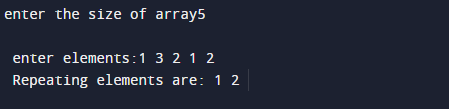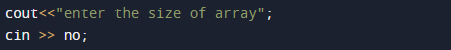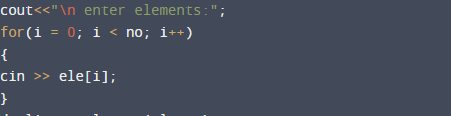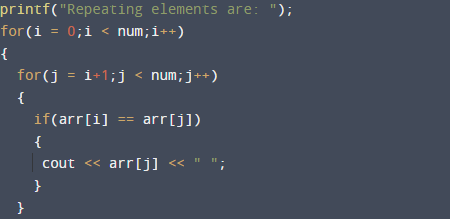# C++ Programs to Find Duplicate Array Element

In this tutorial you will learn about the C++ Programs to Find Duplicate Array Element and its application with practical example.

In this tutorial, we will learn to create a c++ program that find the Duplicate Array Element using c++ programming.

## Prerequisites

Before starting with this tutorial we assume that you are best aware of the following C++ programming topics:

• Operators.
• looping statements.
• Basic input/output.
• Basic c++ programming
• For Loop.
• Array.
• Function.

What Is Array ?

An Array is a collection of similar data type containing  collection of elements (values or variables), each identified by one Array index value, Array is a data structure that  hold  collection of elements.

## C++ Programs to Find Duplicate Array Element.

In this program ,we will find duplicate elements in a Array .First of all user will be prompted to enter a the size or Array and after that user will give elements and we will find the repeating elements in a given Array. Let see the code for finding duplicate values.

## OutputIn the above program, we have first declared and initialized a set variables required in the program.

• no = it will hold size of array
• ele = it will hold a value of array.
• i & j =  for iteration.
• arr= it will hold a value of array.
• num= it will hold total number of array value.

And  in the next statement user will be prompted to enter size of a Array and value of Array.which will be assigned to variable ‘no’ and in ‘ele’ respectively.andNow ,loop through the size given  by user and take all values supply by user in ele variable.Afterword pass this value to function.and within the function in each  iteration we will compare values of array  to find the occurrence of a similar elements and each matching condition we print the matched values .This process  will continue until loop ends within the function, and at last we will print the total number occurrence of a Duplicate values.

In this tutorial we have learn about the C++ Programs to Find Duplicate Array Element and its application with practical example. I hope you will like this tutorial.